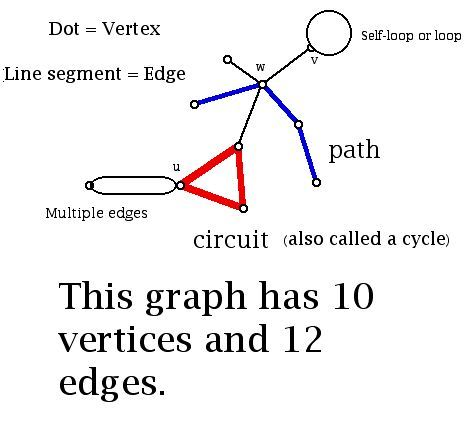Visibility for a Simple Polygon

Prepared by:

Joseph Malkevitch
Department of Mathematics and Computer Studies
York College (CUNY)
Jamaica, New York

email:

malkevitch@york.cuny.edu

web page:

http://york.cuny.edu/~malk

Euclid's Elements, the most enduring book in the history of mathematics, deals predominately with metric properties of geometric figures - area, lengths, angles, etc. In the last 100 years much more attention is being paid to other properties of geometric figures. These properties concern more "combinatorial" properties of geometrical figures. Properties such as convexity and visibility (see glossary at the end for terms that are not defined here and which may be helpful in your work) and ideas related to graph theory (Appendix II) have been pursued. In this microworld of problems I will concentrate on properties related to simple polygons.

A polygon is simple if its sides meet only at vertices. Edges of a simple polygon don't intersect themselves. We will think of a polygon as a collection of rods. A simple polygon divides the plane into the points in the interior of the polygon, on the polygon, and the exterior of the polygon. A set X is convex if whenever two points of X, P and Q are joined by a line segment, all of the points of the segment are points of X. Figure 1a shows a simple convex hexagon (6-gon), and Figure 1b shows a 7-gon which is not simple.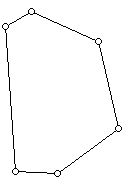Figure 1 (a) Convex hexagon (6-gon)Figure 1 (b) Non-simple 7-gon

For a variety of reasons we will allow more than three of the vertices of a simple polygon to be on a straight line, but we will require that no three consecutive vertices of the simple polygon be collinear (lie on a line).

Thus, we will not allow the situation in Figure 2a but do allow the polygon in Figure 2b.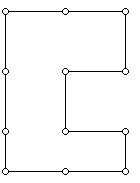Figure 2a A polygon with three consecutive vertices on a lineFigure 2b There are three vertices on a line but these vertices are not consecutive.

The polygons in Figure 2 are special because all of their angles are either 90 degrees or 270 degrees. Such polygons are known as rectilinear or orthogonal polygons.

Two vertices or interior points u and v of a simple polygon P are visible from each if the segment uv does not contain any points of the exterior of P.

A lovely question originally posed by Victor Klee involved the number of vertex guards for a simple polygon. The idea was to study the number of guards stationed at vertices of a polygon who could "see" all of the polygon and its interior. It is known that floor (n/3) guards are sometimes necessary and always sufficient to guard an n-sided simple polygon. Floor x is the largest integer less than or equal to x. Thus, floor (8/3) =2. What the result just stated means is that there is an n-sided simple polygon P for which floor (n/3) vertex guards are needed to see all of P and its interior and that for any n-sided simple polygon one never needs more than floor (n/3) guards. There is no known polynomial time algorithm for finding the minimum number of vertex guards to see all of a simple polygon and its interior.

Here is a simple hexagon which requires 2 guards.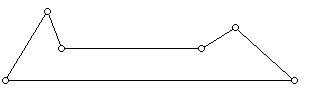Figure 3 A hexagon which requires 2 vertex guards

Problem 1:

Use the diagram in Figure 3 to show how to construct polygons with 3s sides (s=3, 4, ... ) which need s guards.

Art Gallery Theorem (Klee, Chvatal, Fisk)

Floor (n/3) guards located at vertices are sometimes necessary and always sufficient to guard a simple n-sided polygon.

Comment: This problem was posed by Victor Klee, first answered by Vaclav Chvatal and Steve Fisk provided a particularly appealing and simple proof of the result. Fisk's proof relies on an interesting idea in its own right - being able to triangulate a simple polygon.

A triangulation of a simple polygon P is the graph (i.e. diagram with dots and line segments) obtained by using a collection of edges which join existing vertices of the polygon, to subdivide the interior of polygon P into triangles.

While it seems obvious that any simple n-gon can be triangulated, giving a rigorous proof is not that easy unless one has seen the ideas before. (It turns out that it is not the case that every non-self-intersecting non-convex polyhedron can be tetrahedralized, so the analogue of what can be done in the plane cannot be accomplished in 3-dimensional space.) By a triangulated polygon I will mean a graph drawn in the plane where the infinite (unbounded) face is a simple polygon and all of the other faces are 3-gons (triangles). Figure 4 shows a polygon with 11 sides which has been triangulated.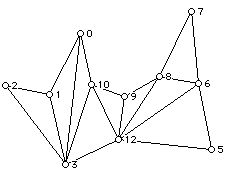Figure 4 Triangulated 11-gon

Problem 2

a. Verify that if P is a simple n-gon then a triangulation of P has (n-2) interior triangles.

b. How many edges are necessary to triangulate an n-sided (n at lest 3) simple polygon?

Problem 3

a. The graph of a triangulated polygon has at least two vertices of valence (degree) 2.

b. For every n (n at least 4) there is a triangulated n-gon with exactly two vertices of degree 2 (valence 2).

c, Are there simple polygons U for every n (3 or more) such that there is a unique way of adding diagonals to U to obtain a triangulated polygon?

Comment: Two triangulations of a simple polygon are different if they use different diagonal (an edge joining two vertices of the polygon which only includes interior points of the polygon) edges.

Given a triangulated polygon considered as a graph, we can write down the set of degrees that can occur.

For example, given the triangulated 7-gon in Figure 5 we have the degrees 2, 2,2, 3, 4, 4, 5.Figure 5

Problem 4

Given a non-negative set of integers D when will these integers be the degrees (valences) of some triangulated polygon?

Comment: A necessary condition of this set of numbers is that there be at least two 2's.

Given a simple polygon P in the plane we can write down for each vertex the number of vertices of the polygon P that are visible from each vertex v of P. For example consider the labeled hexagon H in Figure 6. We will not include a vertex being visible from itself in reporting the counts of the number of vertices visible from a given vertex.Figure 6 A labeled hexagon

For polygon H, the number of vertices visible from u is 2, from w is 4, from x is 4, from y is 2, from z is 4 and from t is 4. Were we to draw a graph V(P) whose vertices are equinumerous to those of the polygon P and where two vertices are jointed if they are visible from each other, the numbers just reported would be the valences or degrees of V(P). The graph V(P) of P is often referred to as the visibility graph of P. V(H) for the hexagon in Figure 6 has degrees 4, 4, 4, 4, 2, 2.

In general the visibility graph of a polygon need not be planar. This is easy to see since if P is a convex polygon with 5 or more vertices, then the visibility graph of G is the complete graph on 5 vertices, that is, a graph with 5 vertices where every vertex is joined to every other vertex.

Problem 5

Verify that a simple polygon with n vertices is convex if and only if the degrees of its visibility graph are all (n-1).

Problem 6

What are the sets of integers that can arise as the degrees of the visibility graph of a simple polygon?

Problem 7

Is there some relationship between the sets of integers that can arise as degrees of a triangulated polygon and the set of degrees that can arise for the vertices of a visibility graph for a simple polygon?

Many of these questions can be specialized to many types of polygons. We have already mentioned rectilinear polygons but there are many other such types. Whenever one can find a criterion whereby two things that are taken to be the same can be seen as different from some point of view, mathematical progress is made. For example, every convex polygon has the property that it has a point from which the whole polygon can be viewed. However, there are many non-convex polygons which have such a point. These are known as the starshaped polygons. Among the kinds of polygons that have been investigated are monotone, vertically convex, staircase, etc. Also, many of these questions can be looked at for equilateral polygons. Perhaps in this situation "better" results are obtainable.

Problem 6

a. Can you find a simple equilateral hexagon which requires 2 vertex guards?

b. Can you find a simple equilateral polygon with 3s sides (s at least 2) which requires s vertex guards?

References

Goodman, J.., and J. O'Rourke, (eds.), Handbook of discrete and computational geometry, CRC press, 2010.

O'rourke, J., Art gallery theorems and algorithms, Oxford University Press, Oxford, 1987.

Sack, J. R., and J. Urrutia, (Eds.). Handbook of computational geometry, Elsevier, 1999.

Appendix I

Glossary:

Collinear points

A set of points which lie on a single line is called collinear.

Concurrent lines

A set of lines which pass through a single point is called concurrent.

Convex

A set of points X is convex if whenever P and Q are points of X the points of the line segment joining P and Q, PQ, are also in X

Convex hull

Given a set X in the plane the convex hull of X consists of the intersection of all convex sets which contain X. (The intersection of two sets is the set of points in both sets.)

Equiangular polygon

A polygon is equiangular if all of its angles have the same measure.

Equilateral polygon

A polygon is equilateral if the length of its sides are all equal.

Exterior and interior of a set of a polygon in the plane

The diagram below shows a simple polygon P. A special case of what is known as the Jordan Curve Theorem states that the points of the plane are points of P andlie in the interior of P or the exterior of P.Graph

A graph is a geometric diagram consisting of points called vertices some of which may be joined together by line segments.

Comment: For a brief primer of graph theory see Appendix II.

Polygonalization

If P is a set of points in the plane, a polygonalization of P is a simple polygon whose vertices are exactly the points of P.

Star-shaped

A set of points X is star-shaped if X has a point P such that for every point of X the segment PX contains no points of the exterior of X. X is said to be visible from P.

Visible

Point P in X is visible from point Q in X if the line segment PQ contains no point exterior to X.

Appendix II

Diagrammatic introduction to graph theory: